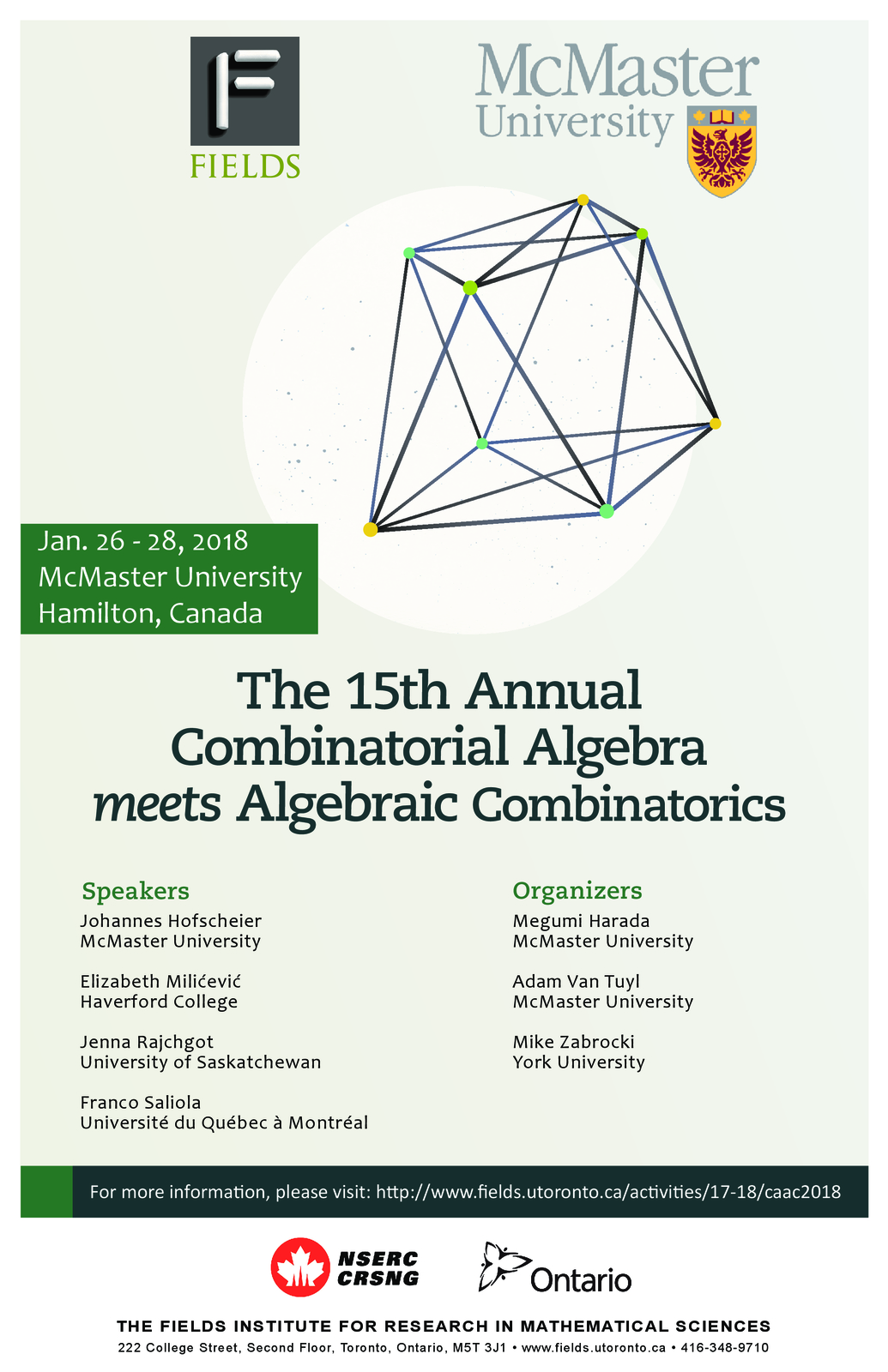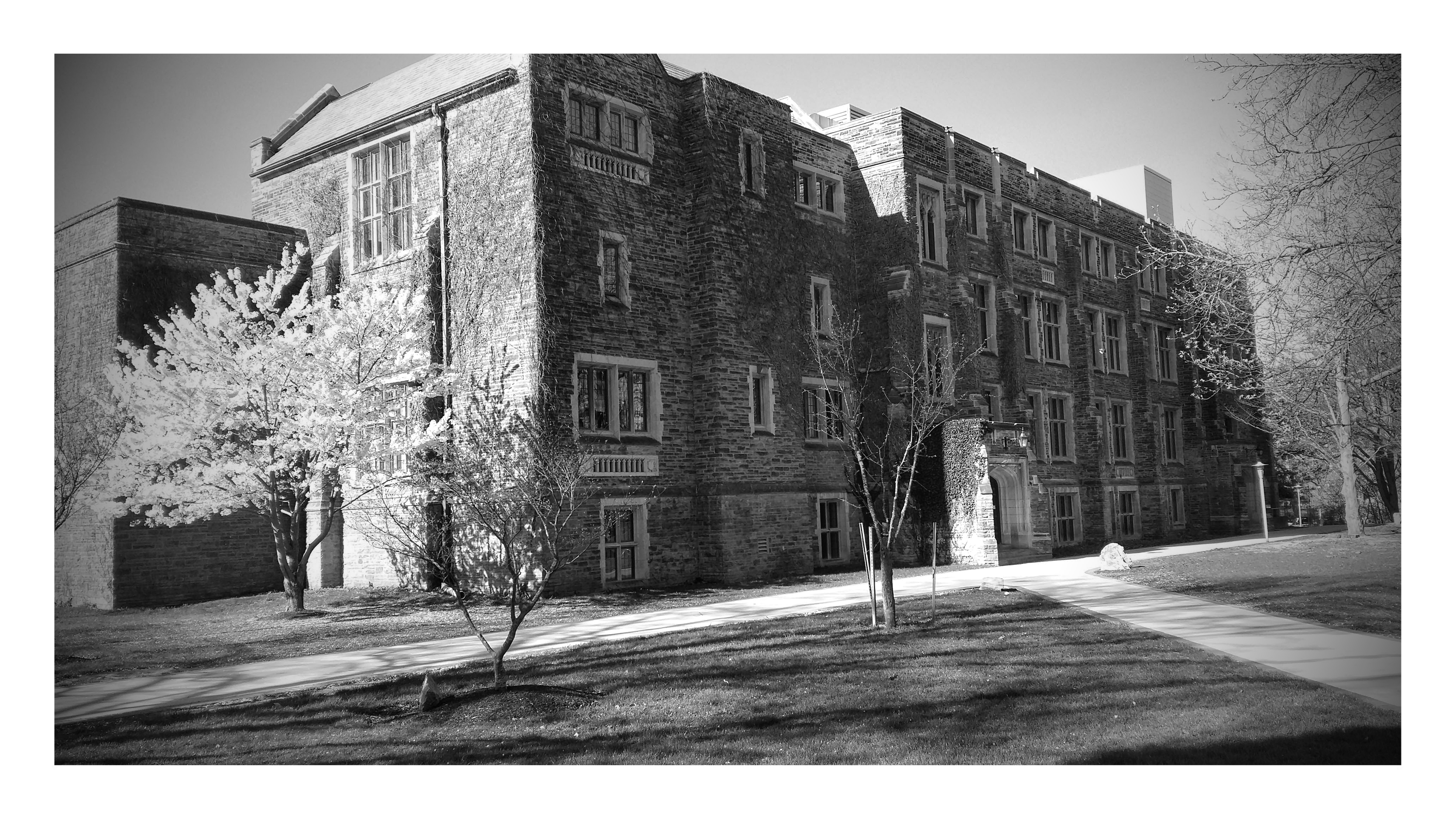# Fifteenth Annual Meeting January 26 - 28, 2018 TITLES and ABSTRACTS# Titles and Abstracts

Francois Bergeron: Structure of generic modules of diagonal harmonic polynomials, and elliptic Hall algebra

Laura Colmenarejo: A minimaj-preserving crystal on ordered multiset partitions

We provide a crystal structure on the set of ordered multiset partitions, which recently arose in the pursuit of the Delta Conjecture. This conjecture was stated by Haglund, Remmel and Wilson as a generalization of the Shu e Conjecture. Various statistics on ordered multiset partitions arise in the combinatorial analysis of the Delta Conjecture, one of them being the minimaj statistic, which is a variant of the major index statistic on words. Our crystal has the property that the minimaj statistic is constant on connected components of the crystal. In particular, this yields another proof of the Schur positivity of the graded Frobenius series of the generalization Rn;k due to Haglund, Rhoades and Shimozono of the coinvariant algebra Rn. The crystal structure also enables us to demonstrate the equidistributivity of the minimaj statistic with the major index statistic on ordered multiset partitions. This is a joint work with Georgia Benkart, Pamela E Harris, Rosa Orellana, Greta Panova, Anne Schilling and Martha Yip.

Nursel Erey: Regularity of powers of $$2K_2$$-free graphs

Let $$S= k[x_1,\dots ,x_n]$$ be the polynomial ring over a field $$k$$ and let $$G$$ be a graph with vertices $$x_1,\dots, x_n$$. The edge ideal $$I(G)$$ of $$G$$ is generated by the monomials $$x_ix_j$$ where $$\{x_i, x_j\}$$ is an edge of $$G$$. It is well-known that if $$I$$ is a graded ideal in $$S$$, then the Castelnuovo-Mumford regularity of $$I^s$$ is a linear function in $$s$$ for $$s\gg 0$$. In this talk, we describe such linear function for edge ideals of some graphs which have no induced $$2K_2$$.

Johannes Hofscheier:Lattice simplices of bounded degree

In this talk, I will present recent joint work with Akihiro Higashitani on a "moduli"-approach for the study of lattice simplices of bounded degree s. More precisely, we introduce a topology on the space of lattice simplices of bounded degree together with a compactification by relating simplices to certain subgroups of the real euclidean space. Using Chabauty-Pontryagin duality, we can show that these subgroups form only finitely many maximal families. We present a classification of these maximal families for the bound s = 2 and conclude the talk with a description of the $$h^*$$-vectors of degree 2 lattice simplices.

Joel Kamnitzer: Crystals and monodromy of Bethe vectors

Crystals are certain graphs which give a combinatorial picture of representations of semisimple Lie algebras. Given two crystals, there is a canonical way to define a crystal structure on their product. This definition is non-commutative and it leads to the action of an interesting group, called the cactus group. This cactus group is the fundamental group of the moduli space of genus 0 real curves. Thus, crystals give us covers of this moduli space. We will explain how these covers arise from a purely algebraic source: the monodromy of eigenvectors for Bethe algebras.

Shu Xiao Li: Theta maps for combinatorial Hopf algebras

Stembridge generalized Schur's $$Q$$ functions to enriched $$P$$-partitions and peak algebra. It later appears that the $$Q$$ functions and peak algebra are odd Hopf subalgebra of symmetric functions and quasi-symmetric functions respectively. We will give a strategy to find the odd Hopf subalgebra of any combinatorial Hopf algebra. The maps from symmetric functions to $$Q$$ functions and from quasi-symmetric functions to peak algebra, called Theta maps, are combinatorial Hopf morphisms and have their own interests. We are going to develop a general theory for theta maps for many families of combinatorial Hopf algebras. In particular, we will describe Theta maps for the Malvenuto-Reutenaur Hopf algebra whose images are potentially generalizations of $$Q$$ functions and peak algebra.

Kuei-Nuan Lin: Edge ideals of oriented graphs

We start with the definitions of the weighted oriented graph, $$D$$, and the edge ideal, $$I(D)$$, associated to the D. We will then talk about why we are interested in this type of ideals. We provide several equivalent conditions for the Cohen-Macaulayness of $$I(D)$$. As an application, we give a complete characterization for the Cohen-Macaulayness of $$I(D)$$ when the underlying (undirected) graph of $$D$$ is obtained from an arbitrary graph by adding a whisker at every vertex. This is joint work with T. Ha, S. Morey, E. Reyes, and R. Villarreal.

Elizabeth Milićević: Flag Varieties and Alcove Walks

Flag varieties play a central role in the intersection of algebraic geometry, representation theory, and algebraic combinatorics. The points in this variety are defined as increasing chains of subspaces in a vector space, but these points also have a convenient combinatorial description given by taking a walk among the alcoves in a Euclidean geometric model for the flag variety. Because flag varieties and their generalizations can be quite large, it can be unwieldy to study the points individually, and so we often collect them into orbit of various groups. We will explain how folding the alcove walks in the geometric model provides a means for visualizing the decomposition of the flag variety into orbits of certain groups. The goal of this talk will be to explain precisely how these algebraic, geometric, and combinatorial stories coincide, primarily by drawing lots of pictures in concrete examples. No specialized background required.

Martha Precup: The cohomology of abelian Hessenberg varieties

Hessenberg varieties are subvarieties of the flag variety with important connections to representation theory, algebraic geometry, and combinatorics. These varieties have gained recent attention due to a conjecture of Shareshian and Wachs relating the chromatic quasisymmetric function of the incomparability graph of a unit interval order to the dot action representation on the cohomology of an associated regular semisimple Hessenberg variety. In this talk, we will discuss an inductive formula for the Betti numbers of certain regular Hessenberg varieties called abelian Hessenberg varieties. Using a theorem of Brosnan and Chow, this formula yields an inductive description of the dot action representation in this case.

Jenna Rajchgot : On the algebra generated by three commuting matrices

In the early 1960s, Gerstenhaber proved that the algebra generated by two commuting $$d\times d$$ matrices has vector space dimension at most $$d$$. The analog of this statement for four or more commuting matrices is false. The three matrix version remains open.

After providing some history and context, I'll translate this three commuting matrix statement into an equivalent statement about certain maps between modules, and prove that this commutative-algebraic reformulation is true in special cases. I'll end with some combinatorial questions about plane partitions, which, if answered, would settle the three matrix analog of Gerstenhaber's theorem for other infinite families of examples.

This is joint work with Matthew Satriano.

Franco Saliola : A Murnaghan-Nakayama Rule for Quantum Cohomology of the Flag Manifold

I will present a general rule for multiplying a quantum Schubert polynomial by a quantum power sum polynomial. This is achieved by relating the structure constants for the multiplication of a quantum Schubert polynomial by a hook quantum Schur polynomial with the structure constants for the (classical) multiplication of a Schubert polynomial by a hook Schur polynomial.

This is joint work with C Benedetti, N Bergeron, L Colmenarejo and F Sottile.

Mayada Shahada: A combinatorial method using the verbal and marginal properties of associative algebras

In this talk, we will explain our new method in proving a close relationship between the factor algebras by the terms of the canonical ascending central series $$F^{(n)}(A)$$ of an associative algebra A and the terms of the canonical descending series $$A^{(n)}$$. Specifically, the finiteness of codimension of some $$F^{(n)}(A)$$ implies the finiteness of dimension of A(n+1). We also show that the converse holds whenever $$A$$ is finitely generated. While this is not true for arbitrary algebras A, we do show that the finiteness of dimension of $$A^{(n+1)}$$ implies, at least, the finiteness of codimension of $$F^{(3n-1)}(A)$$. These results are analogous to the results of Schur, Baer and Hall obtained for groups.

Our developed method in proving these results is quit di↵erent from that used to prove the original theorems of Schur, Baer and Hall. Our approach begins with describing $$A^{(n+1)}$$ and $$F^{(n)}(A)$$, respectively, as the verbal and marginal subspaces of $$A$$ corresponding to a certain polynomial. This ap- proach ended up being very interesting and even opened a wide door for further research.

Jonathan Toledo: Strong persistence property for monomial ideal

An ideal $$I$$ has the strong persistence property if $$\bigl(I^{k+1}\colon I\bigr)=I^k$$ for $$k\geq 1$$. In this talk we study this property for some families of ideals. In particular, we prove that the monomial ideals whose minimal set of generators has degree two have the strong persistence property. We show that a simple hypergraph has the strong persistence property if and only if at least one of its connected components has the strong persistence property. Also, we prove that an unmixed Konig simple hypergraph without 4-cycles has the strong persistence property. We introduce the concept of weighted ideal and furthermore we show that a monomial ideal $$I$$ has the strong persistence property if and only if its weighted ideal $$I_w$$ has the strong persistence property. We prove the last result for the persistence property. Finally, we introduce and study the symbolic strong persistence.

Tianyuan Xu : Classification of Coxeter groups with finitely many elements of $$\mathbf{a}$$-value 2

In 1985, Lusztig defined a function $$\mathbf{a}: W\rightarrow\mathbb{N}$$ for any Coxeter group $$W$$ by using the Kazhdan-Lusztig basis of the Hecke algebra of $$W$$. The $$\mathbf{a}$$-function has important connections with the cell representation theory of $$W$$ and its Hecke algebra, but is usually difficult to compute directly. However, it is known that the set $$E_1=\{w\in W:\mathbf{a}(w)=1\}$$ consists exactly of the non-identity elements of $$W$$ with a unique reduced word, and that $$E_1$$ is finite if and only the Coxeter diagram of $$W$$ satisfy certain conditions. In this talk, we describe a similar classification, in terms of Coxeter diagrams, of all Coxeter groups $$W$$ for which the set $$E_2=\{w\in W:\mathbf{a}(w)=2\}$$ is finite. We will show that the elements of $$E_2$$ are fully-commutative in the sense of Stembridge, and our main tools for the classification include Viennot's heaps and the generalized star operations on Coxeter groups.

Last Updated: Dec. 12, 2017